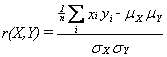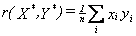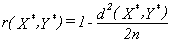Distance and Correlation
Steve Borgatti, Boston College

The purpose of a measure of similarity is to compare two lists of numbers (i.e. vectors), and compute a single number which evaluates their similarity. Most measures were developed in the context of comparing pairs of variables (such as income or attitude toward abortion) across cases (such as respondents in a survey). In other words, the objective is to determine to what extent two variables co-vary, which is to say, have the same values for the same cases.

One problem with comparing two variables is that they may not be measured on the same scale. For example, suppose we are interested in comparing the temperature of one city with the temperature of a nearby city, across a hundred years. Clearly, we expect some relationship between the temperatures. But even if the relationship is absolutely perfect, we don't necessarily expect to see the same numbers. For instance, if one city is in Texas and the other is in Mexico, it may be that one set of temperatures is measured on a Fahrenheit scale, while the others are in Centigrade. Even if the temperatures are both measured in Centigrade, it may be that the thermometers are calibrated differently, so that one reads consistently higher than the other. Consequently, in comparing two temperature variables, we would want to allow for or control for differences in scale.

The general principle is that a measure of similarity should be invariant under admissible data transformations, which is to say changes in scale. Thus, a measure designed for interval data, such as the familiar Pearson correlation coefficient, automatically disregards differences in variables that can be attributed to differences in scale. If you recall, all valid interval scales, applied to the same objects, can translated into each other by a linear transformation. This means that to see how similar two interval variables are, you must first do away with differences in scale by either standardizing the data (this is what the correlation coefficient does), or by trying to find the constants m and b such that the transformed variable mX+b is as similar as possible to Y, and then reporting that similarity (this is what the r-square measure of regression does). Likewise, a measure designed for ordinal data should respond only to differences in the rank ordering, not to the absolute size of scores. A measure designed for ratio data should control for differences due to a multiplicative factor.

Euclidean Distance

The basis of many measures of similarity and dissimilarity is euclidean distance. The distance between vectors X and Y is defined as follows:In other words, euclidean distance is the square root of the sum of squared differences between corresponding elements of the two vectors. Note that the formula treats the values of X and Y seriously: no adjustment is made for differences in scale. Euclidean distance is only appropriate for data measured on the same scale. As you will see in the section on correlation, the correlation coefficient is (inversely) related to the euclidean distance between standardized versions of the data.

Euclidean distance is most often used to compare profiles of respondents across variables. For example, suppose our data consist of demographic information on a sample of individuals, arranged as a respondent-by-variable matrix. Each row of the matrix is a vector of m numbers, where m is the number of variables. We can evaluate the similarity (or, in this case, the distance) between any pair of rows. Notice that for this kind of data, the variables are the columns. A variable records the results of a measurement. For our purposes, in fact, it is useful to think of the variable as the measuring device itself. This means that it has its own scale, which determines the size and type of numbers it can have. For instance, the income measurer might yield numbers between 0 and 79 million, while another variable, the education measurer, might yield numbers from 0 to 30. The fact that the income numbers are larger in general than the education numbers is not meaningful because the variables are measured on different scales. In order to compare columns we must adjust for or take account of differences in scale. But the row vectors are different. If one case has larger numbers in general then another case, this is because that case has more income, more education, etc., than the other case; it is not an artifact of differences in scale, because rows do not have scales: they are not even variables. In order to compute similarities or dissimilarities among rows, we do not need to (in fact, must not) try to adjust for differences in scale. Hence, Euclidean distance is usually the right measure for comparing cases.

Correlation

The correlation between vectors X and Y are defined as follows:where μX and μY are the means of X and Y respectively, and σX and σY are the standard deviations of X and Y. The numerator of the equation is called the covariance of X and Y, and is the difference between the mean of the product of X and Y subtracted from the product of the means. Note that if X and Y are standardized, they will each have a mean of 0 and a standard deviation of 1, so the formula reduces to:Whereas euclidean distance was the sum of squared differences, correlation is basically the average product. There is a further relationship between the two. If we expand the formula for euclidean distance, we get this:But if X and Y are standardized, the sums Σx2 and Σy2 are both equal to n. That leaves Σxy as the only non-constant term, just as it was in the reduced formula for the correlation coefficient. Thus, for standardized data, we can write the correlation between X and Y in terms of the squared distance between them:Note: The original source for this page is: http://www.analytictech.com/mb876/handouts/distance_and_correlation.htm. Minor changes to the original formatting have been applied.